Name:    Quiz 3.1-3.5

Multiple Choice
Identify the choice that best completes the statement or answers the question.

Find the unit rate.

1.

\$12.40 for 4 gallons
 a. \$3.50 per gallon c. \$3.30 per gallon b. \$2.80 per hour d. \$3.10 per gallon

2.

At 4:19 P.M., you have 118 megabytes of MP3 songs. By 4:34 P.M., you have 958 megabytes. What is the download rate in megabytes per minute?
 a. 63.9 MB per min c. 56 MB per min b. 24.7 MB per min d. 7.9 MB per min

Find the slope of the line.

3.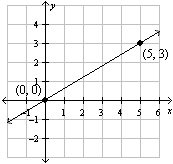a. 3 c.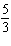b. 5 d.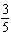4.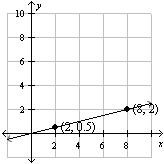a. 2 c.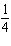b. 8 d.5.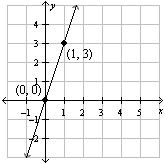a. 6 c. 1 b.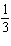d. 3

Write a proportion to find how many points a student needs to score on the test to get the given score.

6.

Test worth 60 points; test score of 60%
 a.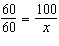c.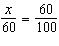b.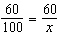d.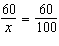7.

Test worth 250 points; test score of 94%
 a.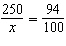c.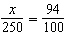b.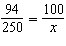d.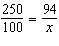Use the table to write a proportion.

8.

 Race 1 Race 2 Meters 40 120 Seconds 7 s
 a.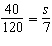c.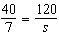b.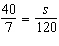d.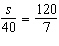Solve the proportion using multiplication.

9.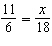a. x = 33 c. x = 36 b. x = 37 d. x = 32

10.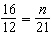a. n = 28 c. n = 31 b. n = 15.8 d. n = 25

11.

There are 40 fluid ounces of juice in 2 bottles of your favorite juice. How many fluid ounces are there in 9 bottles?
 a. 220 fluid ounces c. 180 fluid ounces b. 200 fluid ounces d. 360 fluid ounces

12.

You are planning a party. Your friend tells you how much she paid for five party grab bags. How much will it cost you to buy nine of the grab bags for your party?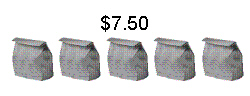a. \$12.00 c. \$21.00 b. \$67.50 d. \$13.50

Solve.

13.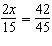a. x = 28 c. x = 7 b. x = 14 d. x = 21

Numeric Response

Solve.

1.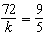2.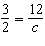1.

Describe a way you can use multiplication to decide whether two ratios form a proportion.

Tell whether the two rates form a proportion.

2.

15 feet in 25 minutes; 21 feet in 42 minutes